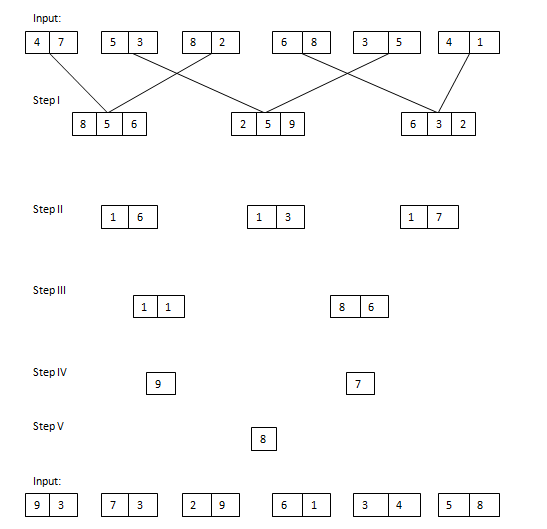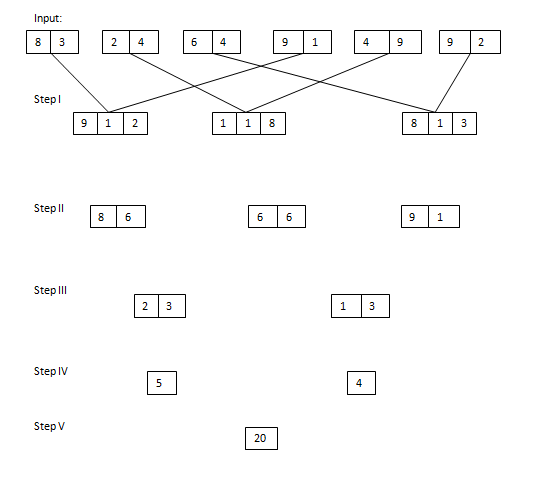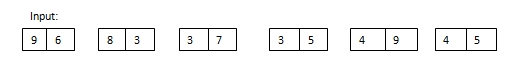# Reasoning – New Pattern Input Output Questions Day-2

Reasoning – New Pattern Input Output Questions Day-2:

Dear Readers, We all knew that IBPS has introduced some new pattern Reasoning Questions in the Examinations. Many of our followers were requesting us to provide Practice Questions based on the new pattern, so for that we have started providing input and output reasoning questions. Kindly follow us regularly and make use of it, if you have any suggestion kindly use the comment section below.

[WpProQuiz 705]

### Click “Start Quiz” to attend these Questions and view Solutions

Directions (1-5): Study the following information carefully and answer the given questions.

A number arrangement machine arranges two digit numbers into a typical manner. Each step gives output taking input from the previous step. The following is an illustration of Input and rearrangement. Using the illustration answer the question given below.1. Which of the following represent difference of the first digit of the third number and third digit of the first number in step I of the given input?
1. 1
2. 2
3. 5
4. 7
5. 8
1. If each digit in the step II is halved and then added, then what will be the final sum?
1. 8.5
2. 12.5
3. 17
4. 14
5. None of these
1. If the value ‘4.5’ is subtracted from the final output, then what will be the resultant value?
1. 18
2. 12
3. 8
4. 4
5. None of these
1. Which of the following represent the first digit of the second number and second digit of the first number in step II?
1. 4, 1
2. 7, 1
3. 1, 4
4. 2, 4
5. None of these
1. What is the difference of two numbers obtained in step III?
1. 37
2. 29
3. 41
4. 18
5. None of these

Directions (6-10): Study the following information carefully and answer the given questions.

A number arrangement machine arranges two digit numbers into a typical manner. Each step gives output taking input from the previous step. The following is an illustration of Input and rearrangement. Using the illustration answer the question given below.1. What is the sum of two numbers obtained in step III?
1. 41
2. 35
3. 54
4. 63
5. None of these
1. If each digit in the step II is subtracted with the value “5” and then added, then what will be the final sum?
1. 17
2. 21
3. 9
4. 13
5. None of these
1. If the value ‘5^2’ is subtracted from the final output, then what will be the resultant value?
1. 6
2. 5
3. 15
4. 21
5. None of these
1. Which of the following combination represent the second digit of the second number and third digit of the first number in step I of the given input?
1. 4, 7
2. 1, 8
3. 7, 9
4. 9, 1
5. 1, 9
1. Which of the following represent the difference between the first digit of the second number and second digit of the first number in step II?
1. 0
2. 4
3. 3
4. 1
5. None of these
• #### Reasoning Input Output New Pattern Questions – Day-1

4 1 vote
Rating